## What is the Internal Rate of Return?

The process of valuing companies or assets involves calculating the return on investment on multiple future cash flows. The internal rate of return (IRR) helps calculate the annual return for future streams of cash flows. The IRR is the discount rate that makes the value of future cash flows equal to the initial investment. In other words, IRR is the discount rate that makes the net present value (NPV) of all future cash flows equal to zero.

## Key Learning Points

• The internal rate of return or IRR for short is a discount rate used in corporate finance which makes the value of future cash flows equal to the initial investment made
• IRR equals discount rate where NPV (net present value) equals zero
• Excel has an IRR function to calculate the internal rate of return
• IRR is an investment appraisal technique and is useful to help managers decide which project is viable
• An IRR above the cost of financing for a project is preferable because the project is generating a return above the cost required to run it

## IRR Explained:

Suppose you are investing \$124.3 today and you expect to receive the following cash flows for the next 3 years. What is your rate of return on this investment?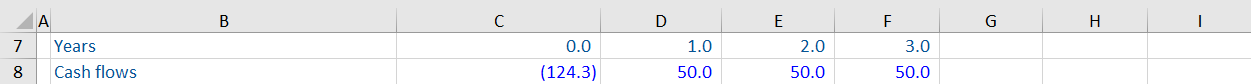One way to calculate the annual returns is to calculate the present value of future cash flows iteratively and add them. Do this using a range of discount rates until the total matches the original investment. Here are the results of a few such iterations: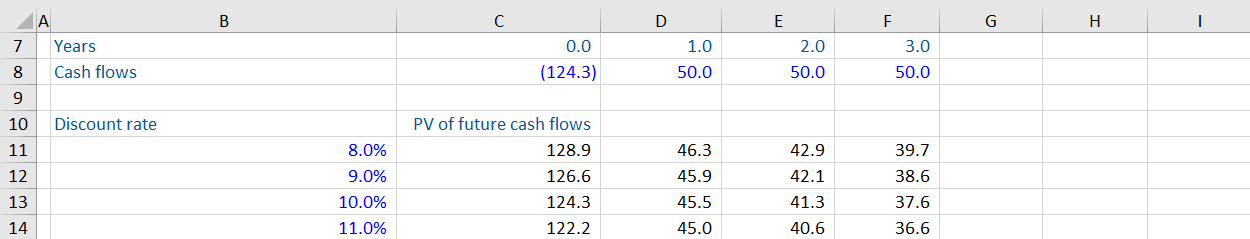Using a discount rate of 8% and 9% results in the present values of future cash flows being higher than the original investment. However, at a discount rate of 10%, the present value of the returns equals \$124.3. And that is the internal rate of return (IRR) for this investment – the annual return is 10%, and the discount rate that would give an NPV of zero is 6%.

## Calculating IRR in Excel

Thankfully, calculating IRR does not have to be so tedious. Using the IRR formula in Excel, you can easily calculate the annual returns on a future stream of cash flows. Here is an example.

Calculate the annual return for the below cash streams. Note that a negative value denotes a cash outflow for the investor.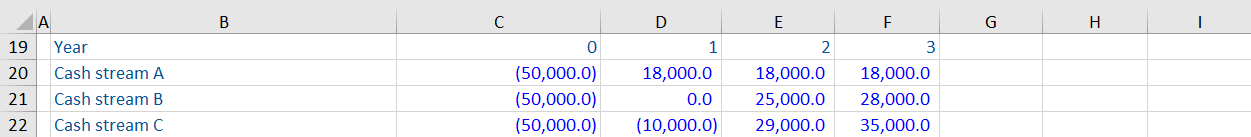Using the IRR function in Excel, you can get the returns for each of these streams as follows: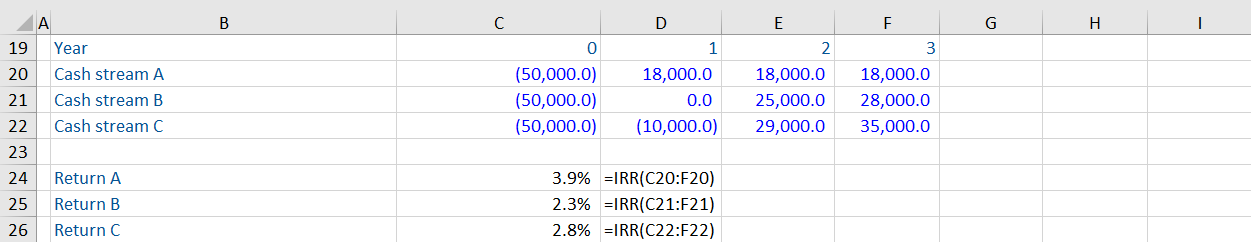The IRR function makes the calculation simpler and allows easy comparison of different cash streams. Here, cash stream A represents the highest annual return.

## Using IRR with NPV

The (NPV) calculates the difference between the present value of cash outflows and cash inflows. As the discount rate increases, the present value of future cash inflows decreases. Theoretically, there is a stage where the discount rate gets so high that the NPV of a project becomes zero. As mentioned above, IRR is the discount rate where the NPV is 0. Let us understand this with an example.

Continuing with the previous example, let us calculate the NPV for each of the cash flows using the IRR as the discount rate. We do these calculations using the NPV function in Excel.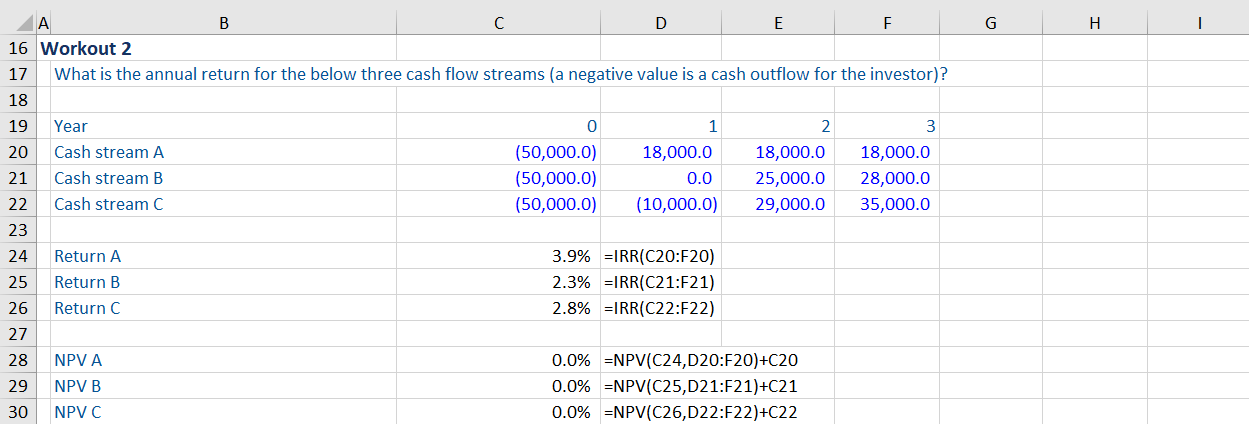As you can see, for all three projects, if you use the IRR as a discount rate for calculating the NPV, the result is 0.

## IRR, NPV & the Cost of Financing

The calculations used above have implications for determining the cost of financing a venture. The IRR should be higher than the cost of financing as it results in a positive net present value. Look at the example below.

Using the IRR and NPV, decide whether you would invest in the following project: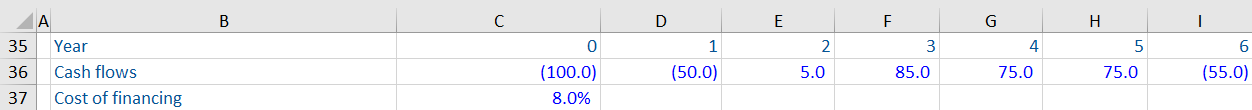Using the IRR and NPV formulas, you get the following: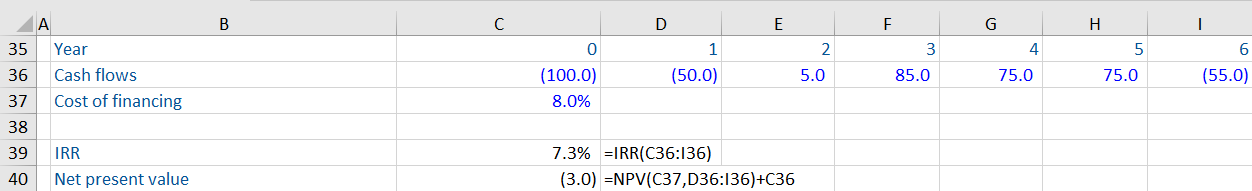As expected, because the cost of financing (8%) is higher than the annual returns (7.3%) from the project, the NPV is negative. If you invest in this project, you will end up losing cash.

Let us try the same calculations by reducing the cost of financing to 7% (below the IRR).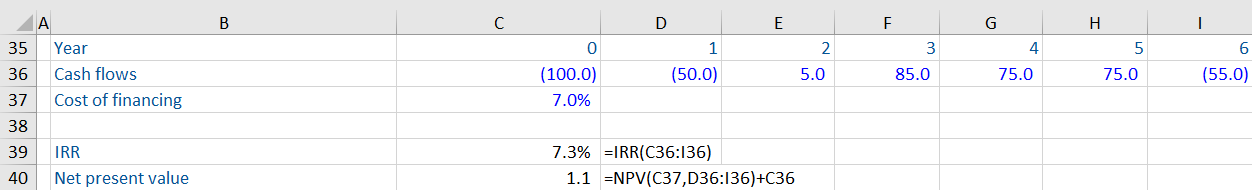In this scenario, the NPV is a positive number, indicating the investment is viable and will return more than was invested.